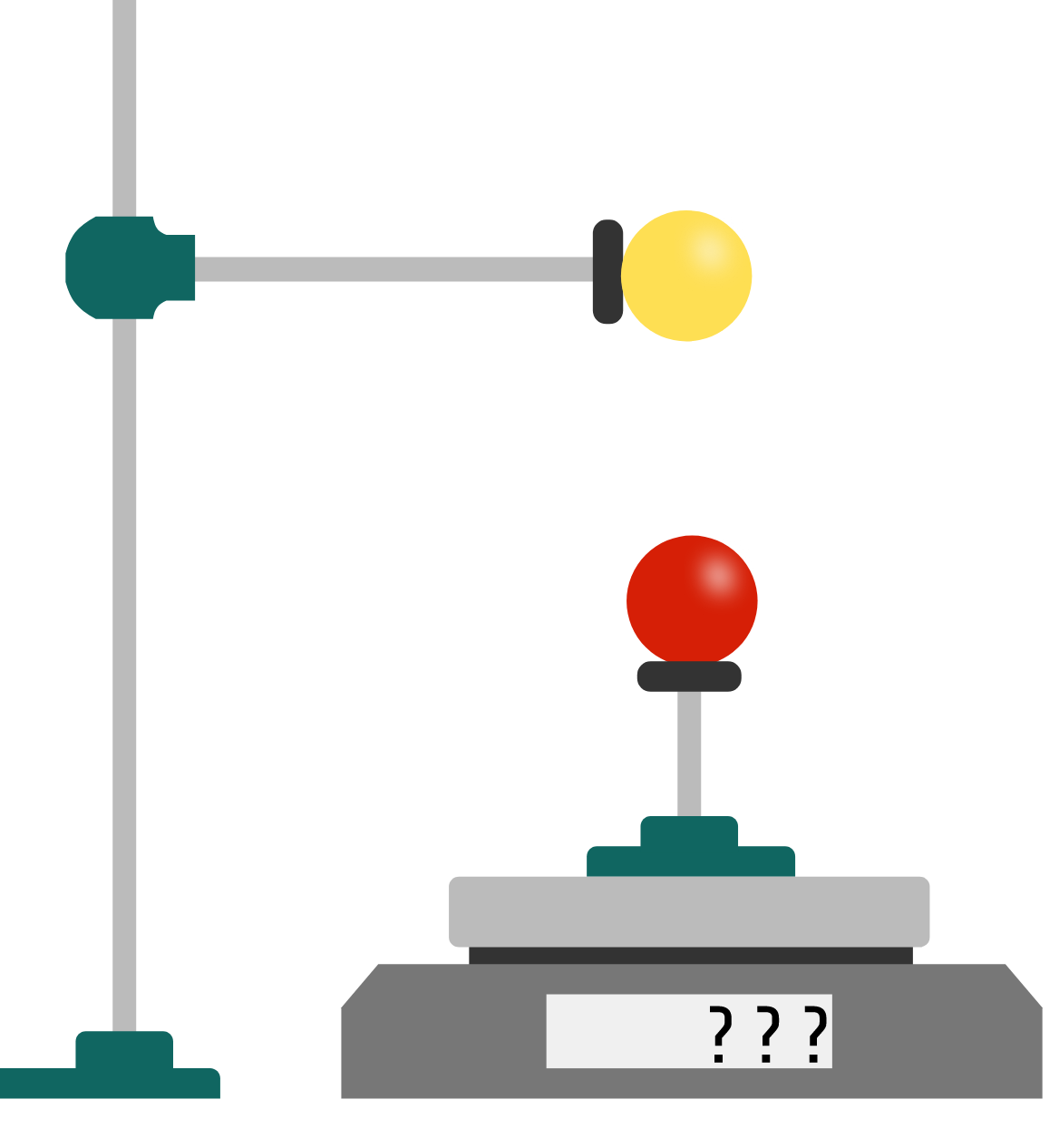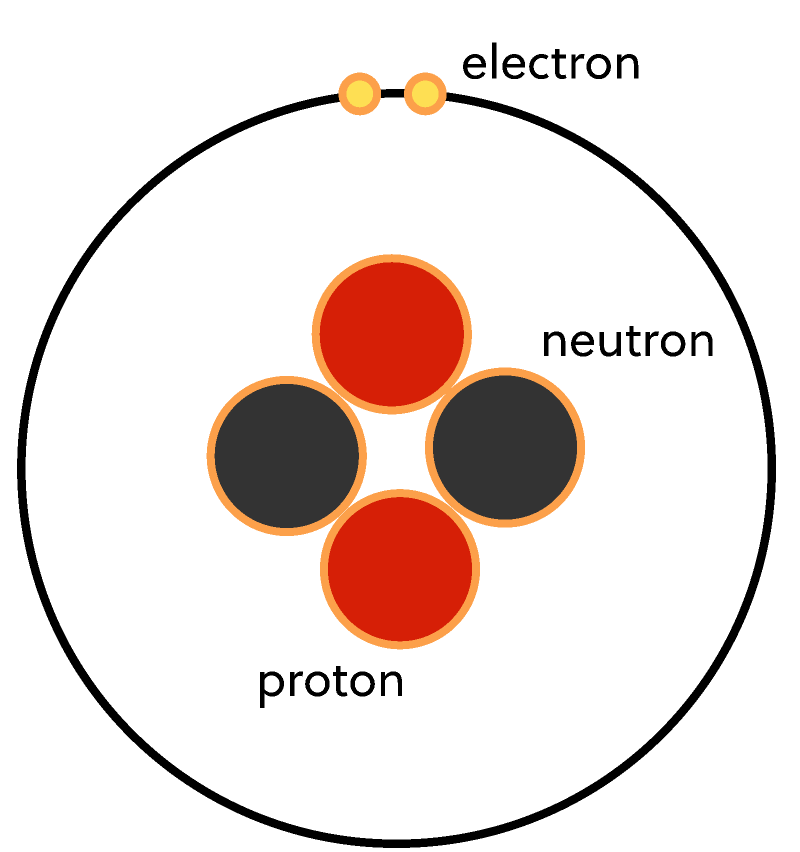Electricity and Magnetism

The experiments in the last few explorations show that matter can become electrically charged. That's because matter comprises "positive" protons and "negative" electrons. After losing or gaining electrons or protons, neutral objects gain a net charge, which subjects them to electric forces, but we haven't explored how strong these electric forces are.

We're in a similar position to the "natural philosophers" who pondered the mysteries of electricity in the $1700$s. They knew how to separate charge and move it around but didn't yet have a model that predicts the forces their charges would feel.This changed in $1782$ when Charles-Augustin de Coulomb carefully measured the forces between charges and found they could be described with a simple rule, known today as Coulomb's law.

In this quiz, we'll follow in his footsteps... with a slight update to his equipment.

Coulomb's Law

In our experiments with tape strips, we saw that the electric force is spooky — it acts between objects that aren't touching. Coulomb realized that he could use a common piece of laboratory equipment to measure the strength of the force: a sensitive balance.We can re-create his experiment with a modern digital scale. First, we'll place a positively charged metal ball on an insulating stand and place that on the scale.

Next, we'll take a negatively charged ball and clamp it to a support, directly above the first ball. What "weight" will the scale show?

Coulomb's Law

The scale gives us an indirect way to measure the amount of electric force, $F_e,$ produced by the electric charge on the two balls. In his experiment, Coulomb hoped to discover a rule that would predict $F_e,$ starting from the amount of charge on each ball.

To measure the electric force directly, we should "zero" the scale when the balls are uncharged — this way, the scale reading will only report the contribution from the electric force.

We can place some positive charge on the ball that's on the scale, and adjust the (positive) electric charge $Q_1$ on the ball above the scale to see what effect it has on the force.

Try playing with the charge in this simulated experiment. When the charge on one of the balls doubles, what happens to the strength of the electric force?

Coulomb's Law

This shows that the electric force $F_\text{e}$ is directly proportional to the charge $Q_1$ on the top ball.

But there's nothing special about the top ball: if we adjust the charge $Q_2$ on the bottom ball instead, we'll observe the same relationship.

This means that if we increased the charge on either ball by a factor of, say, $100,$ while keeping the other constant, the force on both balls also increases by the same factor of $100.$

So, the electric force $F_e$ must be proportional to the product of the two charges. We can write this as $F_\text{e} \propto Q_1 \times Q_2.$

Coulomb's Law

Unlike Newton's law for gravity, the electric force is sometimes attractive, and sometimes repulsive. It turns out that the relationship we just found, $F_e \propto Q_1 \times Q_2,$ also gives us a convenient mathematical trick to predict whether we'll see an attractive or repulsive force.

First, we need to assign negative charges a negative sign, and positive charges a positive sign. For example, if a proton has charge $+q,$ then an electron would have charge $-q.$

How does the sign of the product $Q_1\times Q_2$ relate to the direction of an electric force?

Hint: to get started, consider case when $Q_1$ is a negative charge and $Q_2$ is also a negative charge. Is the electric force attractive or repulsive?

Coulomb's Law

Select one or more

In the last chapter, we saw that charged objects feel stronger electric forces when they're closer to each other, so this dependence on charge is not the end of the story.

Coulomb used his torsion balance to measure the electric force between metal balls, varying the distance $d$ between their centers.

We can do the same with our version of the experiment, by moving the clamped ball up and down above the ball on the scale.

Try adjusting the distance between the two balls. If you double the distance, what happens to the force?

Coulomb's Law

By moving the balls to different positions, it's also possible to work out the direction of the force.

Bringing together the results of these experiments, we find the following:

The electric force between two stationary point charges $Q_1$ and $Q_2$ that are separated by a distance of $r$ is $F=k_\text{e} \frac{ Q_1 Q_2}{r^2},$ and it's directed along the line connecting the point charges.

If $F$ is negative, then the force points towards the other charge; otherwise, it points away from the other charge.

This relationship is called Coulomb's law, and the constant $k_\text{e}$ is called the Coulomb constant.

Coulomb's Law

We've defined the Coulomb constant $k_\text{e}$, but what is its value?

The number depends on the units we use to measure electric charge. In the SI systems of units, charge is measured in Coulombs, with the symbol $\si{\coulomb}$.

This unit represents a lot of charge — a typical lightning strike delivers $\approx\SI{15}{\coulomb}$ from cloud to ground.

When electric force is measured in Newtons $(\si{\newton}),$ Coulomb's constant has the value $k_\text{e}=\SI[per-mode=symbol]{9e9}{\newton\metre\squared\per\coulomb\squared},$ and in our experiments with the tape, we were probably dealing with excess charges around $\SI{E-7}{\coulomb}.$

Using Coulomb's law, roughly how strong were the forces between the two pieces of tape when we hold the pieces of tape $\SI{10}{\centi\metre}$ apart?

Coulomb's Law

So, net charges of just over $1/\num{10000000}$ of a Coulomb on either tape strip give rise to a Newton, a force big enough to hold up an apple (no joke intended).

Turning back to the particle picture, net charge comes from the imbalance of protons and electrons. Indeed, the charge on an object is simply proportional to the number of protons minus the number of electrons: $Q_\text{net} = +e\times\left(n_p - n_e\right),$ where the charge on a single proton (or minus the charge of an electron) is $+e = \SI{1.6e-19}{\coulomb}.$

Approximately what is the magnitude of $\left(n_p-n_e\right)$ for the tape strips in our experiment?

Coulomb's Law

Though it looks simple, Coulomb's law can already tell us something important about the world — that the protons in the nucleus are bound by immense forces!

All atoms are made of positive protons, negative electrons, and neutral neutrons, but these particles aren't spread evenly through the space inside. The protons and neutrons are packed into a tiny nucleus that's only a few femtometers $\big(\SI[per-mode=symbol]{E-15}{\metre}\big)$ across, which was revealed by atomic physics experiments in the late $19^\text{th}$ century.The structure of a helium atom, containing two protons, two neutrons, and two electrons

The charge on a proton is $\SI[per-mode=pedagogical]{+1.60E-19}{\coulomb}$. To hold together two protons at a distance of $\SI[per-mode=pedagogical]{2E-15}{\metre},$ how strong is the repulsive electric force between them?

Coulomb's Law

On its own, the electric force between two protons would force them apart and blow the nucleus apart — so there must be "nuclear forces" holding them together that are stronger than the electric forces.

Modern physics incorporates four fundamental forces. On the smallest scales, the strong and weak nuclear forces act — but their strength decreases very quickly with distance. Outside the nucleus, these forces disappear, and electric forces are most important.

On much larger scales than atoms, most matter is electrically neutral, containing an equal balance of protons and electrons (counterintuitively, this is a consequence of the strength of electric forces). This cancels out the electric forces, leaving the weakest force, gravity, to bring the neutral atoms together into planets, stars, and galaxies.

Coulomb's law works very well for two point-like charges such as metal spheres. But our world is full of extremely complicated situations containing many charged particles, spread out over complex shapes. To simplify these situations, we need more tools — the first of which is the electric field.

Let's get started.

Coulomb's Law

×